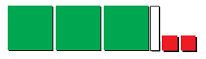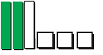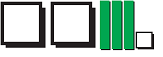# Characteristics of polynomials#### Everything You Need in One Place

Homework problems? Exam preparation? Trying to grasp a concept or just brushing up the basics? Our extensive help & practice library have got you covered.#### Learn and Practice With Ease

Our proven video lessons ease you through problems quickly, and you get tonnes of friendly practice on questions that trip students up on tests and finals.#### Instant and Unlimited Help

Our personalized learning platform enables you to instantly find the exact walkthrough to your specific type of question. Activate unlimited help now!##### Intros
###### Lessons
1. What is a polynomial?
• Review on Variables, Coefficients, and Expressions
• What are Monomials, Binomials, and Trinomials?
• What are the Degree, Leading Term, and Constant term of a polynomial?
• Name polynomials based on degree: Quadratic, Cubic, Quartic, Quintic, etc.
2. How to find the degree of a polynomial?
##### Examples
###### Lessons
1. Determine the number of terms and the name for each of the expression.
1. $- 5{y^2}$
2. $2{x^2} + 4x + 6$
3. $- 7.3$
4. $y^3 + ay + nx - x^2$
2. Find the degree and number of terms for each of the following expression.
1. $2xy - 3{y^2} + 6$
2. $4{a^3} + a$
3. $- 4$
3. Write the expression for each set of the algebra tiles.

1.2.3.4. Peter wants to buy some comic books and magazines. A bookstore sells comic books for $12 each and magazines for$8 each.
1. Write an expression to represent the money Peter needs to spend on both comic books and magazines.
2. How much does Peter need to spend if he wants 9 comic books and 3 magazines?
3. Write a new expression for Peter's expenditure on books if the bookstore raises the price of a comic book to $15 and a magazine to$10.
##### Practice
###### Topic Notes
A polynomial consists of constants, variables and exponents. Polynomials are named based on the number of terms they have. Polynomials with 1 term are called monomials. Those with 2 terms are called binomials, and the ones with 3 terms are called trinomials.